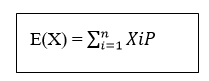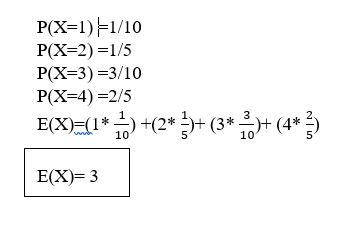### Expected Value

• date 25th June, 2019 |
• by Prwatech |

# Expected Value tutorial

Expected value tutorial: Welcome to Data science tutorials from Prwatech, In this one can learn about expected value in data science and how to calculate it with examples. Before we proceed, we recommend you to go through the previous blog in this series on how to calculate the probability density function in data science.

Are you the one who is looking for the best platform which provides information about what is Expected value? or Are you the one who wants to become an expert Data Scientist? Then you have landed on the right path which provides advanced Data science training from Prwatech having highly skilled expert trainers with 100% best placement.

## What is the Expected Value?

Expected Value in Data Science of a Random Variable is the theoretical mean of the random variable.

It is exactly what you might think it means intuitively: the return you can expect for some kind of action, like how many questions you might get right if you guess on a multiple-choice test.

### Why we need the Expected Value?

Suppose if you take 200 questions multiple-choice test with A, B, C, D as the answers, and you guess all “D”, then you can expect to get 25% right

The calculation behind this kind of expected value is:

The probability (P) of getting a question right if you guess: .25
The number of questions on the test (n)*: 20
P x n = 0.25 x 200 = 50. This is called as Expected Value.

## Expected Value Formula## Expected Value Example

• A stack of cards contains one card labeled with 1, two cards labeled with 2, three cards labeled with 3, and four cards labeled with 4. If the stack is shuffled and a card is drawn, what is the expected value of the card drawn?

Solution:

Let X be the random variable that represents the value of the card drawn. ThenInterested in learning more? Or do you want to become an expert in Data Science? get your Data science advanced course from Prwatech, India’s Leading Data science training institute in Bangalore.

We hope you liked our article on the expected value tutorial. Share your feedback with your comments.

### Quick Support